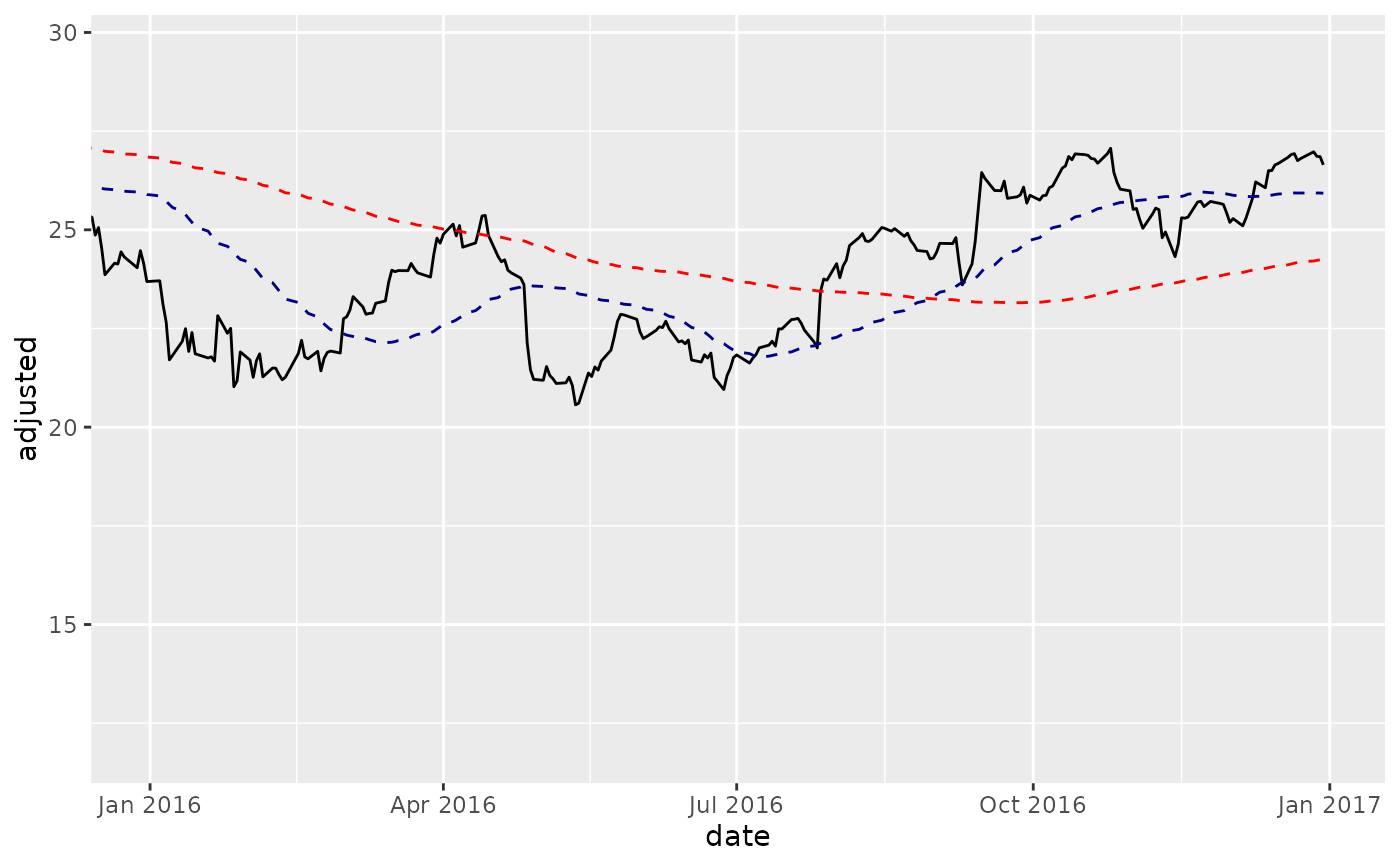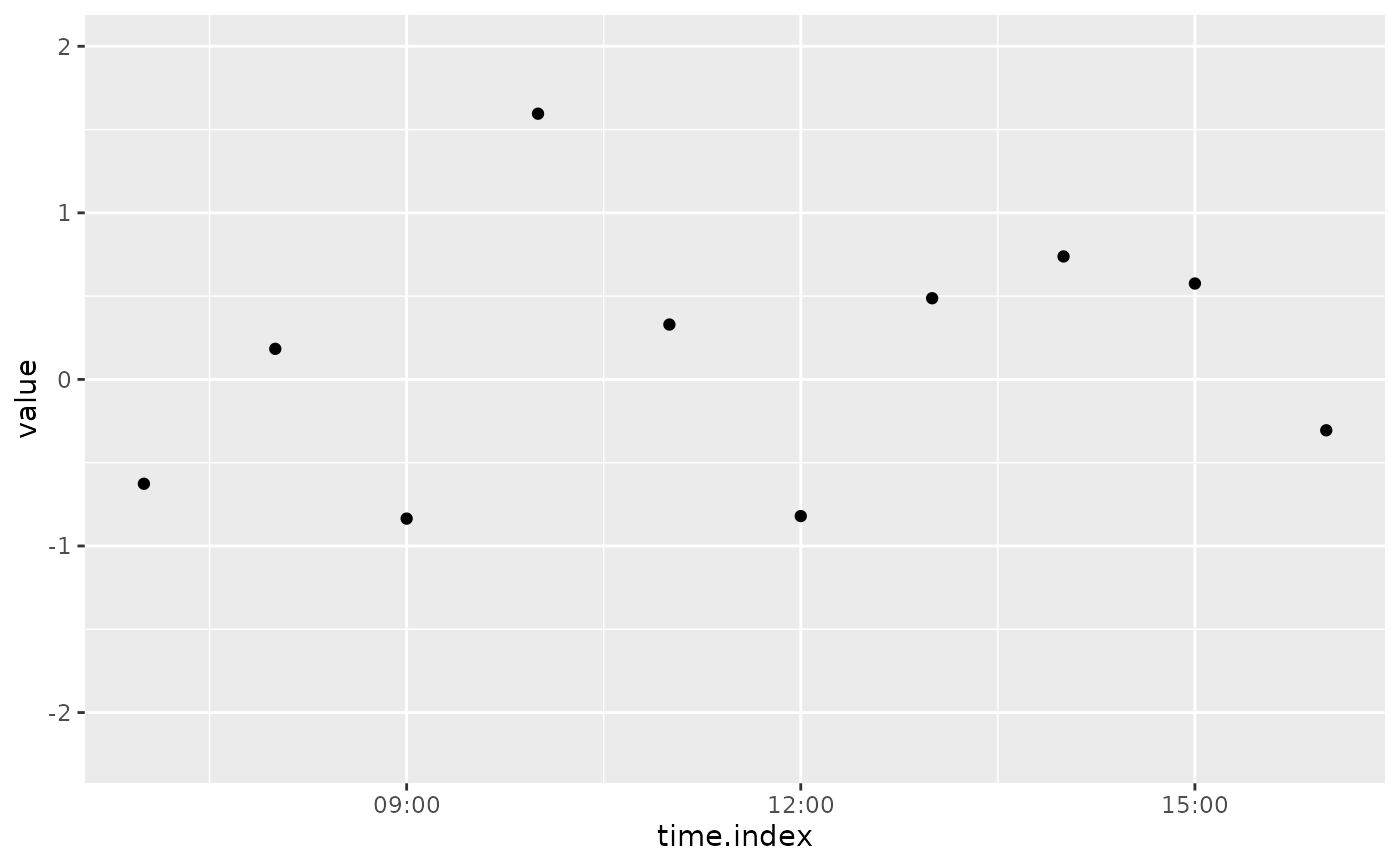Zoom in on plot regions using date ranges or date-time ranges

coord_x_date(xlim = NULL, ylim = NULL, expand = TRUE)

coord_x_datetime(xlim = NULL, ylim = NULL, expand = TRUE)

## Arguments

xlim

Limits for the x axis, entered as character dates in "YYYY-MM-DD" format for date or "YYYY-MM-DD HH:MM:SS" for date-time.

ylim

Limits for the y axis, entered as values

expand

If TRUE, the default, adds a small expansion factor to the limits to ensure that data and axes don't overlap. If FALSE, limits are taken exactly from the data or xlim/ylim.

## Details

The coord_ functions prevent loss of data during zooming, which is necessary when zooming in on plots that calculate stats using data outside of the zoom range (e.g. when plotting moving averages with geom_ma()). Setting limits using scale_x_date changes the underlying data which causes moving averages to fail.

coord_x_date is a wrapper for coord_cartesian that enables quickly zooming in on plot regions using a date range.

coord_x_datetime is a wrapper for coord_cartesian that enables quickly zooming in on plot regions using a date-time range.

ggplot2::coord_cartesian()

## Examples

# Load libraries
library(tidyquant)
library(dplyr)
#>
#> Attaching package: ‘dplyr’
#> The following objects are masked from ‘package:xts’:
#>
#>     first, last
#> The following objects are masked from ‘package:stats’:
#>
#>     filter, lag
#> The following objects are masked from ‘package:base’:
#>
#>     intersect, setdiff, setequal, union
library(ggplot2)

# coord_x_date
AAPL <- tq_get("AAPL", from = "2013-01-01", to = "2016-12-31")
#> Registered S3 method overwritten by 'tune':
#>   method                   from
#>   required_pkgs.model_spec parsnip
AAPL %>%
ggplot(aes(x = date, y = adjusted)) +
geom_line() +                         # Plot stock price
geom_ma(n = 50) +                     # Plot 50-day Moving Average
geom_ma(n = 200, color = "red") +     # Plot 200-day Moving Average
# Zoom in
coord_x_date(xlim = c("2016-01-01", "2016-12-31"),
ylim = c(75, 125))# coord_x_datetime
time_index <- seq(from = as.POSIXct("2012-05-15 07:00"),
to   = as.POSIXct("2012-05-17 18:00"),
by   = "hour")
set.seed(1)
value <- rnorm(n = length(time_index))
hourly_data <- tibble(time.index = time_index,
value      = value)
hourly_data %>%
ggplot(aes(x = time.index, y = value)) +
geom_point() +
coord_x_datetime(xlim = c("2012-05-15 07:00:00", "2012-05-15 16:00:00"))